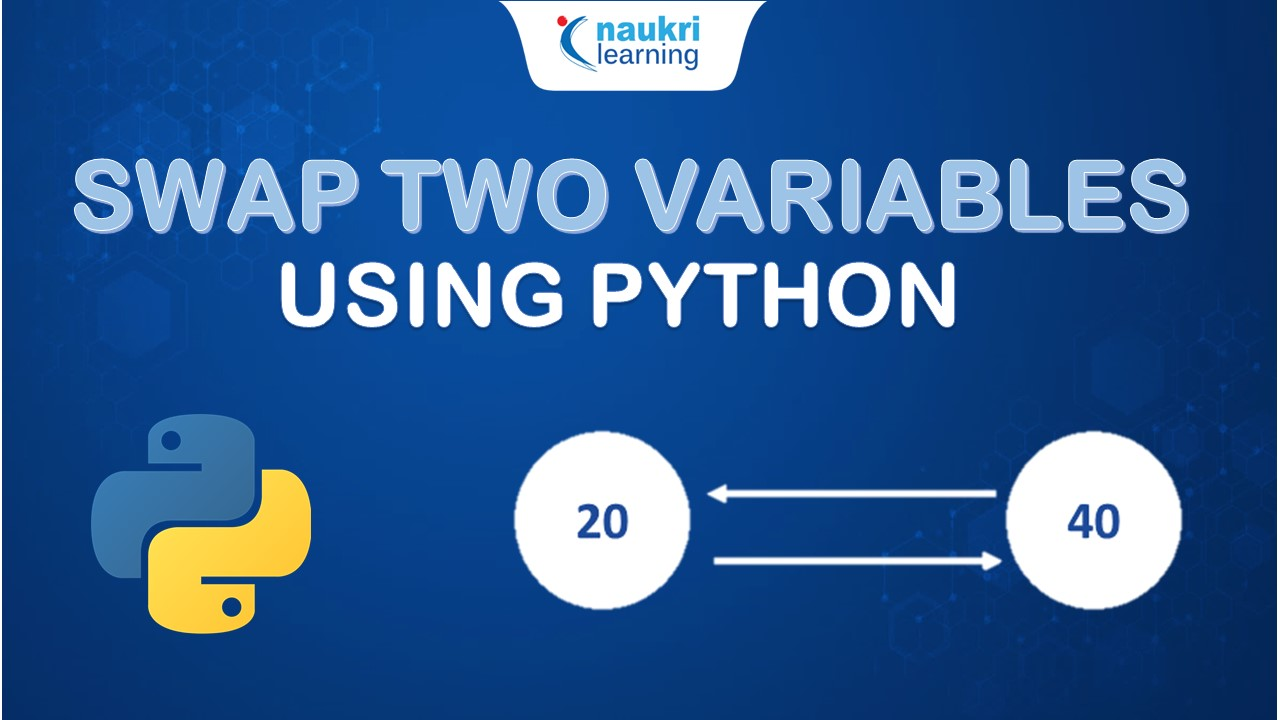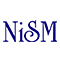Floor Division in Python

# Floor Division in Python

clickHere
Updated on Nov 22, 2022 19:19 IST

Through this article, you will be able to learn the concept of Floor Division in Python. You will understand its use through three methods.

## Introduction

In Python, we can divide any two numbers and round off the resultant to the nearest integer with the help of the Double-Backslash (//) operator that is, Floor Division operator //.

## Working of Floor Division Operator

The // operator is applied to two numerical values first division will be performed and then the result obtained from the division will be rounded down to the nearest integer.

We can use floor division in the program using the following ways:

## Method 1: Floor division using // operator

### 1. Example of / and // with a positive integer

` `
```#Python program illustrating normal division operator result = 15/4 print ("The division of 15 by 4 is:", result)The division of 15 by 4 is: 3.75 #Python to illustrate Floe division using // operator result = 15//4print("Floor division of 15 by 4 is:",result)Floor division of 15 by 4 is: 3Copy code```

In the above code, we can see that when we divide 15 by 4, the result will be 3.75.

When we use Floor division for 15 and 4, we see that the output will be 3 that is, the 3.75 is rounded off to the nearest integer which is 3.

We can use floor division in the program using the following ways:

### 2. Floor Division with Negative Integer

When floor division is performed on a negative integer then the operation will be the same as a division

That is if a dividend is negative, and the divisor is a positive integer, then the quotient will be a negative integer.

If a dividend is negative and the divisor is also a negative integer, then the quotient will be positive. If a dividend is positive and the divisor is a positive integer, then the quotient will be a negative integer

` `
```#Floor division with negative number print("Floor division of -15 and 4 is:" , -15//4)print ("Floor division of -15 and -4 is:" ,-15//-4)print ("Floor division of 15 and -4 is:" ,15//-4)Floor division of -15 and 4 is: -4Floor division of -15 and -4 is: 3Floor division of 15 and -4 is: -4Copy code```

First Case: Dividend = -15, Divisor = 4

-15/4 = -3.75

Hence the output will be rounded off to -4.

Why -4 and not -3?

Because rounding down a negative number means going away from zero. For example, -3.5 is floored down to -4.

Second Case: Dividend = -15 and Divisor = -4

-15/-4 = 3

Hence the output will be rounded off to 3.

Third Case: Dividend = 15 and Divisor = -4

15/-4 = -3.75

Hence output will be rounded off to -4.

### 3. Floor Division on Float Value:

` `
`#Floor division using // operator with float valueprint("Floor division of 9 and 3.5 is", 9//3.5)print ("Floor division of 9.5 and 3.5 is", 9.5//3.5)print("Floor division of 9.5 and 3 is", 9.5//3)Floor division of 9 and 3.5 is 2.0Floor division of 9.5 and 3.5 is 2.0Floor division of 9.5 and 3 is 3.0Copy code`

Python’s Float Division works with Float datatypes as well.

If Floor division is applied on Float datatype then the output will also be Float as seen in the above code examples.

## Method 2. Using math.floor()

A built-in math module in Python provides practical mathematical tools for calculations.

The math.floor feature is one of the built-in capabilities of the math module (). With a numerical input, this function levels the outcome to the closest integer.

` `
```#Importing math moduleimport math #Floor division using math.floor()print(math.floor(7/2)) 3Copy code```

### Method 3. Floor Division in Python Using __floordiv__():

Whenever we use // operator between two objects the __floordiv__() gets called implicitly. Instead, we can directly use __floordiv__() between two objects.

For example:

` `
```#Floor division using_floordiv_() methodprint((15). _floordiv_(4)) 3Copy code```Python Program to Swap Two Variables
In this article, we will learn how to swap two variables using the Python programming language. Here’s the agenda!Support Vector Machines: Python codeString Formatting in Python
In this article we discussed different techniques of string formatting in python like modulo operator, built-in format and f-string.

## Alternative methods for //:

When working with untidy data, for instance, you could occasionally encounter datasets that contain both integers and floats.

In some cases, the / operator will output both floats and integers, giving conflicting results. Therefore, utilizing the / operator will require additional steps to assure a consistent output if your program depends on integers.

There are a few additional methods for changing the quotient of two numbers so that it functions properly with your software. Using various methods can give you more control over the outcomes, as you might not always want to round a value down.

### 1. math.floor():

Math.floor() rounds its argument to the nearest integer to produce the same outcome as the floor division operator.

Regardless of the given data type, math.floor() always outputs an integer, which is a notable difference. Math.floor() is more helpful when working with both integers and floats since it produces a more reliable output.

We have already seen the working of math.floor above.

### 2. math.ceil():

Math.ceil() is an alternative to math.floor() that will always round up to the nearest whole number rather than down.

For Example:

` `
`#Program to illustrate math.ceilprint ("math.ceil() is applied for 15 and 4. The result is:", math.ceil (15/4))math.ceil() is applied for 15 and 4. The result is: 4Copy code`

### 3. int():

You can cast a float datatype to an int datatype for an immediate solution. When a float is passed to int(), the result is an integer with everything after the decimal point is removed.

In the example below,

15/4 = 3.5. However, casting to an integer will remove 0.75.

` `
`#Program to illustrate int()print ("int is applied for 15 and 4. The result is:", int (15/4))int is applied for 15 and 4. The result is: 3Copy code`

#### Precedence of Floor Division Operator:

The precedence of the floor division (//) operator is the same as that of multiplication (*), division (/), and modulo (%).

Example:

As // and * have the same precedence the operator which comes first will be executed first and then the next operator:

` `
`#Program to illustrate precedence of // print (7//2*3)9Copy code`

Step by Step execution:

7//2 = 3

3*3 = 9

Hence the output will be 9 in this case.

Example 2:

` `
`print (7*2//3)4Copy code`

As the * operator comes first multiplication is performed and then floor division.

#### Step-by-Step Calculation:

7*2 = 14

14//3 = 4 (14/3 = 4.666 that will be rounded off to 4)

Hence output will be 4.

## Conclusion

When an integer is required or you need to return the smallest integer that is less than or equal to the input, you typically use the floor division operator (/).

The output will be an integer if the operands are both integers. The result will be a float datatype if either operand is a float.

Although lines with the / operator are idiomatic and easy to read, it may be preferable to use math.floor(), math.ceil(), int(), or round when working with inconsistent and disorganized datasets ().

I hope this article clarified the concept of floor division along with different methods as to how to define it. You can also check out our articles on Python to understand different aspects of the language.

_______________

Recently completed any professional course/certification from the market? Tell us what you liked or disliked in the course for more curated content.

clickHere

This is a collection of insightful articles from domain experts in the fields of Cloud Computing, DevOps, AWS, Data Science, Machine Learning, AI, and Natural Language Processing. The range of topics caters to upski... Read Full Bio

## Trending Data Science CoursesData Science with Python
UDEMY4.2Become a Data Science Professional with IIT Certification in Advanced ProgrammingBecome a Data Science Professional with IIT Certif...
Guvi5.0Become a Data Science Professional with IIT Certification in Advanced ProgrammingBecome a Data Science Professional with IIT Certif...
Guvi5.0Starts 15 Dec

## Top Picks & New Arrivals